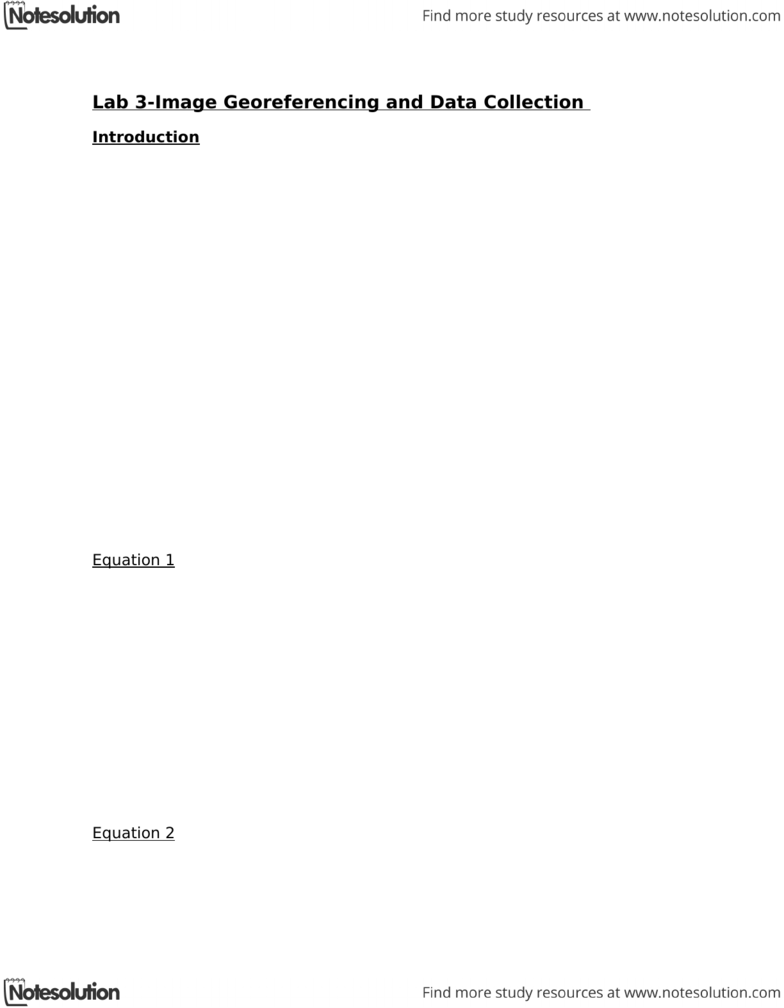Class Notes (1,100,000)
CA (620,000)
York (40,000)
ESSE (70)
Sohn (10)
Lecture

# ESSE 3600 Lecture Notes - Georeferencing, Arcgis, Second Order (Religious)

by

Department
Earth, Space Science and Engineering
Course Code
ESSE 3600
Professor
Sohn

Page:
of 8Lab 3-Image Georeferencing and Data Collection
Introduction
In Lab 3, students were introduced to image georeferencing and data
collection. The purpose of this exercise was to become familiar with the co-
registration process between image data and map vector data using
digitization. Since there was no alignment between image features and map
features, the map had to be rectified by selecting control points.
At first, the data was auto-adjusted using the georeferencing toolbar in
ArcGIS. The vector data was loaded onto the map, and they were two
separate shapefiles. Since the alignment between the map data and the
vector data was not 100% identical, an attempt was made to select fifteen
control points and then fix the vector alignment with the map data. Fifteen
control points were selected and then saved in a View Link table that
contained information about X and Y coordinates, in order to determine the
minimum number of points required for polynomial transformation.
First Order Polynomial Transformation
The equation to determine the minimum number of points for first order
polynomial transformation is as follows:
Equation 1
n=# of control points
To determine the number of control points,
control points=(n+1)(n+2)/2
For a first order polynomial this is,
control points=(1+1)(1+2)/2=(2*3)/2=3
According to the equation, 3 points are required for first order polynomial
transformation.
Second Order Polynomial Transformation
Equation 2
n=# of control points
To determine the number of control points,
control points=(n+1)(n+2)/2
For a second order polynomial this is,
control points=(2+1)(2+2)/2=(3*4)/2=6
According to the equation, 6 points are required for second order polynomial
transformation.
Third Order Polynomial Transformation
Equation 3
n=# of control points
To determine the number of control points,
control points=(n+1)(n+2)/2
For a third order polynomial this is,
control points=(3+1)(3+2)/2=(4*5)/2=10
According to the equation, 10 points are required for first order polynomial
transformation.
RMS Error
The RMS error is the root-mean-squared error of the residual errors. It takes
an average of all the residual errors in the given data. The given data is
shown below in link tables. There were fifteen control points selected.
First Order Polynomial Transformation
Here, RMS is 12.81752. This is highly inaccurate and shows a large error
margin.
Second Order Polynomial Transformation
Here, RMS is 11.4963. This is highly inaccurate and shows a large error
margin, though it is slightly more accurate than the first order
transformation.